Test: Phasor Diagrams

# Test: Phasor Diagrams

Test Description

## 15 Questions MCQ Test Network Theory (Electric Circuits) | Test: Phasor Diagrams

Test: Phasor Diagrams for Electrical Engineering (EE) 2023 is part of Network Theory (Electric Circuits) preparation. The Test: Phasor Diagrams questions and answers have been prepared according to the Electrical Engineering (EE) exam syllabus.The Test: Phasor Diagrams MCQs are made for Electrical Engineering (EE) 2023 Exam. Find important definitions, questions, notes, meanings, examples, exercises, MCQs and online tests for Test: Phasor Diagrams below.
Solutions of Test: Phasor Diagrams questions in English are available as part of our Network Theory (Electric Circuits) for Electrical Engineering (EE) & Test: Phasor Diagrams solutions in Hindi for Network Theory (Electric Circuits) course. Download more important topics, notes, lectures and mock test series for Electrical Engineering (EE) Exam by signing up for free. Attempt Test: Phasor Diagrams | 15 questions in 45 minutes | Mock test for Electrical Engineering (EE) preparation | Free important questions MCQ to study Network Theory (Electric Circuits) for Electrical Engineering (EE) Exam | Download free PDF with solutions
 1 Crore+ students have signed up on EduRev. Have you?
Test: Phasor Diagrams - Question 1

### The voltage applied to a circuit is 100 √2 cos (100πt) volts and the circuit draws a current of 10 √2 sin (100πt + π / 4) amperes. Taking the voltage as the reference phasor, the phasor representation of the current in amperes is

Detailed Solution for Test: Phasor Diagrams - Question 1

Given that

V(t) = 100√2 cos (100πt),

i(t) = 10√2 sin(100π + π/4).

To consider voltage as a reference phasor, The First step would be to make Cosine as Sine by adding 90º to its phase.

V(t) = 100√2 cos (100πt)

= 100√2 sin (100πt + π/2)

i(t) = 10√2 sin(100π + π/4)

Phasor diagram for above two equations can drown as below.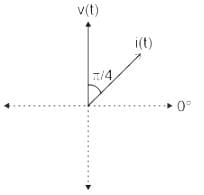As you can see by considering voltage as reference current leg behind by 45º.

So RMS value of current in phasor form is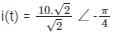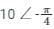Test: Phasor Diagrams - Question 2

### Three currents i1, i2, and i3 meet at a node. if i1 = 10 sin (400t + 60°) A, and i2 = 10sin (400t - 60°) A then i3 =

Detailed Solution for Test: Phasor Diagrams - Question 2

Kirchhoff’s Current Law (KCL): It states that the algebraic sum of currents entering a node or closed boundary is zero.

Mathematically, KCL implies that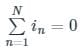Where N is the number of branches connected to the node

And in is the nth current entering or leaving the node.

By this law current entering a node may be regarded as positive and current leaving a node may be regarded as negative or vice-versa.

Hence, the sum of currents entering a node is equal to the sum of the currents leaving the node.

Calculation:

Given that, three currents i1, i2, and i3 meet at a node,

From the above concept,

i1,+ i2, + i3 = 0

or,  i= - (i1 + i2)

Since the current is given in phasor form, hence the addition of current i1 and i2 can be done by using the parallelogram method,

We have,

i1 = 10 sin (400t + 60°) A .... (1)

i2 = 10sin (400t - 60°) A .... (2)

The phasor diagram can be drawn as,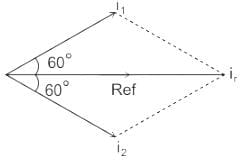By using the parallelogram method,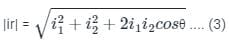Where, it is the resultant current

We have, θ = (60° + 60°) = 120°

From equation (1), (2) & (3),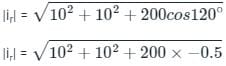= 10

Since i1 & i2 has the same phase angle as well as the magnitude,

∴ ir = 10sin 400t A

Since the sum of all current is zero, hence,

i3 = - ir = - 10sin 400t A

Test: Phasor Diagrams - Question 3

### The phasor diagram of a load is as follows: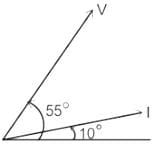Detailed Solution for Test: Phasor Diagrams - Question 3

Types of loads and Phasor Diagrams:

• The load which contains only inductance (L) and not any other quantities like resistance and capacitance in the circuit is called a Pure inductive load.
• In this type of load, the current lags behind the voltage by an angle of 90 degrees.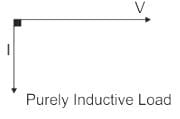Pure capacitor:

• The load which contains only capacitance (C) and not any other quantities like resistance and inductance in the circuit is called a Pure capacitive load.
• In this type of load, the current leads the voltage by an angle of 90 degrees.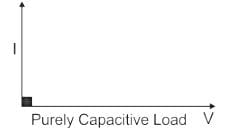• The load which contains only Resistance (R) and Inductance (L) and not any other quantities like capacitance in the circuit is called a RL load.
• In this type of load, the current lags behind the voltage by an angle between 0 to 90 degrees.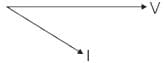• When a pure resistance of R ohms, a pure inductance of L Henry, and a pure capacitance of C farads are connected together in series combination with each other then RLC Seriesload is formed.
• As all the three elements are connected in series so, the current flowing through each element of the load will be the same as the total current I flowing in the load.

Case I

RLC load with inductive reactance more than the capacitive reactance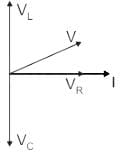Where

VL = Inductor voltage

VC = Capacitor voltage

I = Current

V = Supply voltage

VR = Resistor Voltage

Case II

RLC load with inductive reactance lesser than the capacitive reactance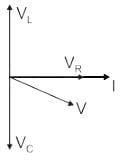So If we observe the phasor diagram between V and I in all cases, RL load or RLC with inductive reactance more than the capacitive reactance (Case II) matches the given phasor.

Test: Phasor Diagrams - Question 4

In phasor representation of an alternating quantity, the sinusoidally varying alternating quantity can be represented graphically by:

Detailed Solution for Test: Phasor Diagrams - Question 4

Concept:

A phasor is a vector representation of a sinusoidal function that has both a magnitude and a phase angle.

Representations:

Rectangular form: z = x + jy

Polar form: z = r ∠ ϕ

Exponential form: z = z e

Comparison:

Time Domain: V(t) = Vm cos (ωt + ϕ)

It represents the voltage as a function of time as shown: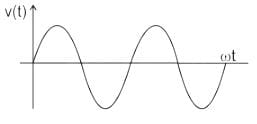Phasor Domain: V = Vm ∠ ϕ

It gives the magnitude and phase and can be represented as: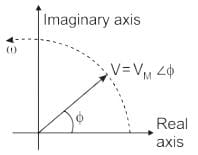Where,

Vm is the magnitude of the voltage function

ϕ is the phase angle of the voltage function

Note:

Power factor = cos ϕ

• The phase angle is negative for lagging power factor
• The phase angle is positive for leading power factor

In the phasor representation of an alternating quantity, the sinusoidally varying alternating quantity can be represented graphically by a straight line with an arrow.

Test: Phasor Diagrams - Question 5

The current in the inductive circuit is 10 A and power factor is 0.866. The phasor representation of current is:

Detailed Solution for Test: Phasor Diagrams - Question 5

Given-

Im = 10 A,

Power factor = 0.866 lagging (∵ inductive circuit)

∴ cosϕ = cos-1(0.866)

∴ ϕ = -30° (∵ lagging power factor)

So that phasor representation of current is given by

I = 10 ∠-30° A

Test: Phasor Diagrams - Question 6

For a pure resistance supplied through a sinusoidal voltage, the phase difference between the voltage and current phasors will be _______.

Detailed Solution for Test: Phasor Diagrams - Question 6

Resistance:

• If the pure resistive element is present in the circuit, then the current is in phase with the voltage. So angle is 0°
• The power factor in this case is unity.

Inductance:

• If the pure inductive element is present in the circuit, then the current lags the voltage by 90 degrees.
• The power factor in this case is lagging.

Capacitance:

• If the pure capacitive element is present in the circuit, then the current leads the voltage by 90 degrees.
• The power factor, in this case, is leading.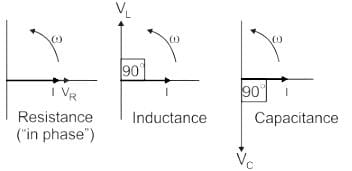Hence the correct option for angle between the current and voltage in pure resistive circuit is zero or 0°.

Test: Phasor Diagrams - Question 7

Three different coils produce a same EMF of 100 V when moved at a constant speed in the magnetic field. The induced EMF in the first coil lags to the induced EMF in the second coil and leads to the induced EMF in the third coil. So, what will be the resultant EMF in the series combination of the three coils?

Detailed Solution for Test: Phasor Diagrams - Question 7

Concept:

Coils induce EMF if moved with constant velocity in the magnetic field in accordance with Faraday's law of electromagnetic induction, and this EMF is also called Motional EMF. This is a basic law of electromagnetism.

Sum of 3 equal phasors at 0°,120°, -120° apart is zero.

Calculations :

Consider, E1 = 100∠0 V,  E2 = 100∠120 V,  E3 = 100∠-120 V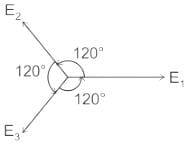Resultant EMF, ER = E1 + E2 + E3

E= 100∠0 + 100∠120 + 100∠-120

ER = 100ej0 + 100ej120+100e−j120

=100[ej0+ej120+e−j120]

=100[1+2cos(120)]

0

Test: Phasor Diagrams - Question 8

A phasor

1. may be a scalar or a vector

2. is a time-dependent quantity

3. is a complex quantity

Which of the above statements are correct?

Detailed Solution for Test: Phasor Diagrams - Question 8

Phasor diagrams are a graphical way of representing the magnitude and directional relationship between two or more alternating quantities.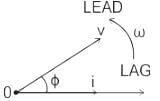Basically, a rotating vector called a Phasor is a scaled line whose length represents an AC quantity that has both magnitude and direction which is frozen at some point in time.

Characteristics:

• It is a vector quantity
• It is a complex quantity
• It is a time dependent quantity
Test: Phasor Diagrams - Question 9

The phase difference between current and voltage in a pure capacitive circuit is:

Detailed Solution for Test: Phasor Diagrams - Question 9

When the Alternating emf is running in the circuit is

e = e0 sin ωt

Current in the inductive circuit is –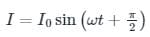• From above it is clear that current leads the voltage by π/2.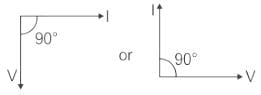Hence

the Phase difference between current and voltage in pure capacitive circuit is 90° or π/2.

Test: Phasor Diagrams - Question 10

The non linear magnetization curve of a practical transformer will introduce _________

Detailed Solution for Test: Phasor Diagrams - Question 10

If the magnetization is non linear in nature then it will cause a saturation in the core and harmonics will be introduced to cause humming sounds.

Test: Phasor Diagrams - Question 11

Which of the following equation correctly represents the exact phasor diagram of transformer?

Detailed Solution for Test: Phasor Diagrams - Question 11

According to the primary and secondary equivalent circuits of a transformer equation stated in option 1 correctly suits with the kirchoff’s voltage law for primary side of a transformer, similarly equation for secondary side can also be written down.

Test: Phasor Diagrams - Question 12

If the phasors are drawn to represent the maximum values instead of the rms values, what would happen to the phase angle between quantities?

Detailed Solution for Test: Phasor Diagrams - Question 12

When phasors are drawn representing the maximum values instead of the rms value, the shape of the diagram remains unaltered and hence the phase angle remains the same.

Test: Phasor Diagrams - Question 13

Usually phasor diagrams are drawn representing?

Detailed Solution for Test: Phasor Diagrams - Question 13

Ammeters and voltmeters are calibrated to read the rms value, hence the phasors are drawn representing the rms values.

Test: Phasor Diagrams - Question 14

The rms value is _________ times he maximum value

Detailed Solution for Test: Phasor Diagrams - Question 14

We know that the rms value is 1/√2 times the maximum value, hence the rms value is 0.707 times the maximum value.

Test: Phasor Diagrams - Question 15

Phasor diagram below is best describing the __________

Detailed Solution for Test: Phasor Diagrams - Question 15

Graph of Ef vs Ff is the open circuit characteristic of the machine.

## Network Theory (Electric Circuits)

23 videos|63 docs|61 tests
Information about Test: Phasor Diagrams Page
In this test you can find the Exam questions for Test: Phasor Diagrams solved & explained in the simplest way possible. Besides giving Questions and answers for Test: Phasor Diagrams, EduRev gives you an ample number of Online tests for practice

## Network Theory (Electric Circuits)

23 videos|63 docs|61 tests(Scan QR code)